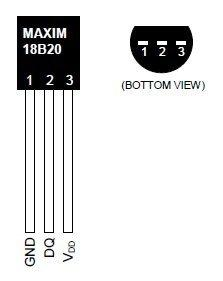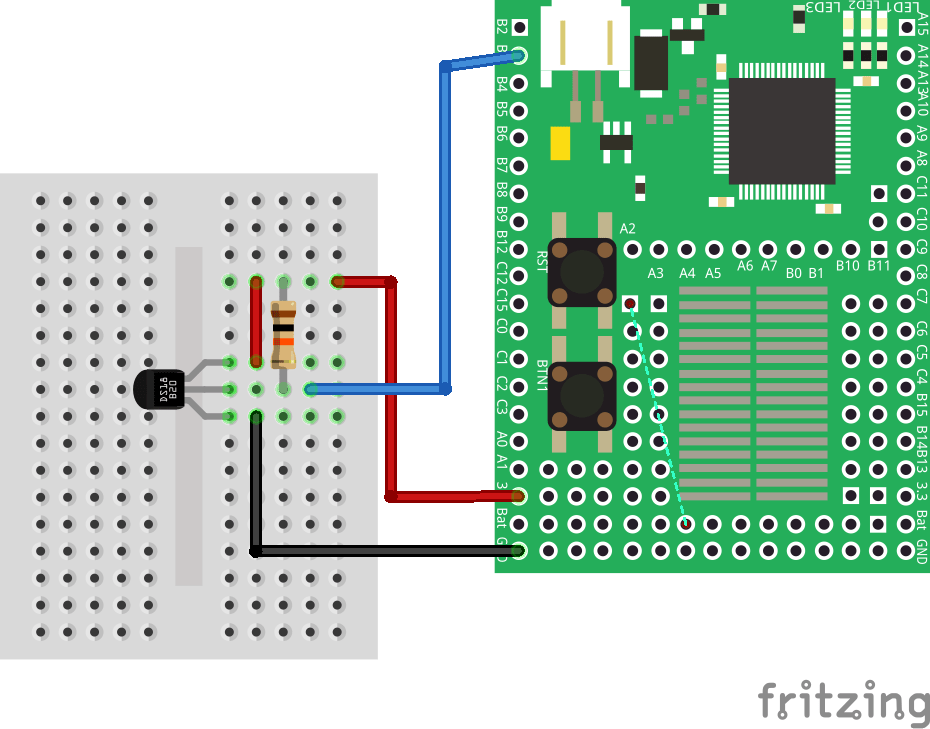# Espruino and DS18B20 temperature sensor

The DS18B20 is a  temperature sensor that can be used in various simple projects.  This part uses the 1 wire  bus and you can connect multiple sensors up to your Espruino.

The part is also relatively low cost and only requires an additional 4k7 pull up resistor. In the example below we shall make a basic example that reads the temperature and outputs via serial and can be verified using the console window in Espruino Web IDE.

Lets look at the parts list

Parts List

Label Part Type
DS1 DS18B20 1-Wire Temperature Sensor
Part1 Espruino
R1 4.7k Ω Resistor

Layout

As always be careful not to get the connections incorrect, you can refer to the pinout for the device below to help . The DS18B20 can be powered by between 3.0V and 5.5V so you can simply connect its GND pin to 0V and the VDD pin to +3v3 from the EspruinoHere is the connection diagram showing how to connect your Espruino to the resistor and sensor.Code

[codesyntax lang=”javascript”]

```require("DS18B20");

var ow = new OneWire(B3);
var sensor = require("DS18B20").connect(ow);
setInterval(function() {
sensor.getTemp(function (temp) {
console.log("Temp is "+temp+" degrees celsius");
});
}, 1000);```

[/codesyntax]

Testing

View the console window in the Espruino Web IDE

Temp is 24.5 degrees celsius
Temp is 24.5 degrees celsius
Temp is 24.5 degrees celsius
Temp is 24.4375 degrees celsius
Temp is 24.5625 degrees celsius
Temp is 25.3125 degrees celsius
Temp is 26.0625 degrees celsius
Temp is 26.75 degrees celsius
Temp is 27.375 degrees celsius
Temp is 27.875 degrees celsius
Temp is 28.3125 degrees celsius
Temp is 28.625 degrees celsius
Temp is 28.9375 degrees celsius
Temp is 29.1875 degrees celsius
Temp is 29.4375 degrees celsius
Temp is 29.625 degrees celsius
Temp is 29.75 degrees celsius
Temp is 29.5625 degrees celsius